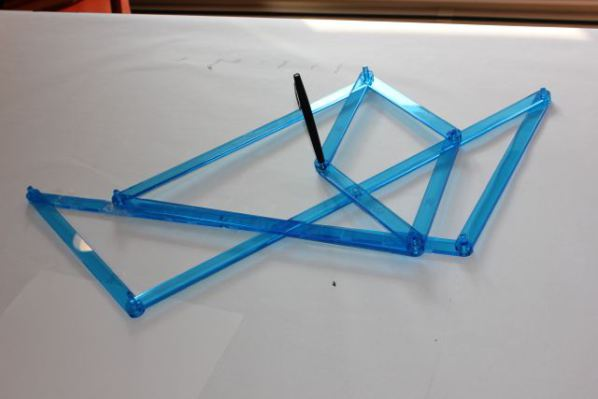### Math Monday: Linkages – Give ’em the Second Degree

by Gareth Branwyn

We’re back after a short hiatus — hope your 2013 is starting out mathematically auspiciously. (And lest the last two digits of the year worry you, note that typically, from a mathematical point of view, the multiplicative factors of a number are more significant than addends that produce the number. After all, every number is 13 plus something, so there’s not much meaning to the fact that 2013 = 2000 + 13. But 13 is not a factor of 2013 = 3 * 11 * 61, so no need for triskadekaphobes to worry.)

This is another installment in our epic Math Monday series on the intricate world of mechanical linkages. See the Linkages series introduction for the MoMath Linkage Kit, an introduction, and general instructions.

So, by now we’ve seen linkages that trace out curves described by fourth degree polynomials, and sixth degree polynomials, and most recently, lines: "curves" of degree one. So, the natural question: is it possible to trace out a curve of degree two? Indeed, it is, and this column gives you a linkage for a parabola. Interestingly, like the Peaucellier linkage for a straight line, this linkage depends on the technique of inversion in a circle. In fact, you should be able to see a copy of the Peaucellier linkage embedded in this one. It’s the most complex linkage in this MoMath series.

Here’s a picture of the completed linkage:A reminder — be careful to cross the two 60-bars as in the above picture. You don’t want the first four bars to form a parallelogram. In other words, you don’t want your linkage to come out looking like this paper model:And finally, here’s the parabola linkage in action, drawing a rather lovely curve:For a real Math Mondays challenge, work out why this linkage produces a perfect parabola. You can also find linkages on the web for the other conic sections: ellipses and hyperbolas. (The linkage for a circle was covered in the very first column: it’s the 1-bar linkage known as a “compass”.)